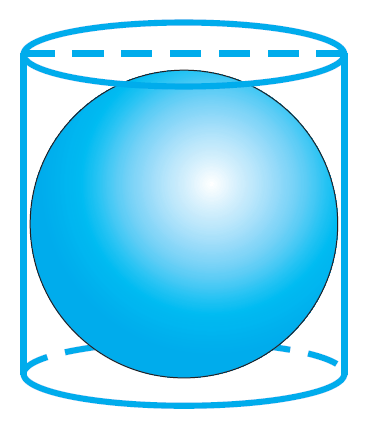# vs.eyeandcontacts.com

## Chapter 13 Surface Areas and Volumes Exercise 13.4

Question 1: Find the surface area of a sphere of radius:
i) 10.5 cm
ii) 5.6 cm
iii) 14 cm

i) Given
Radius of the sphere = 10.5 cm
Surface area of sphere = ?
TSA of sphere = 4πr²
= 4 × 22/7 × 10.5 × 10.5
= 4 × 22 × 1.5 × 10.5
= 1386 cm²

ii) Given
Radius of the sphere = 5.6 cm
Surface area of sphere = ?
TSA of sphere = 4πr²
= 4 × 22/7 × 5.6 × 5.6
= 4 × 22 × 0.8 × 5.6
= 394.24 cm²

iii) Given
Radius of the sphere = 14 cm
Surface area of sphere = ?
TSA of sphere = 4πr²
= 4 × 22/7 × 14 × 14
= 4 × 22 × 2 × 14
= 2464 cm²

Question 2: Find the surface area of a sphere of diameter:
i) 14 cm
ii) 21 cm
iii) 3.5 m

i) Given
Diameter of the sphere = 14 cm
Surface area of sphere = ?
Radius = Diameter/2 = 14/2 = 7 cm
TSA of sphere = 4πr²
= 4 × 22/7 × 7 × 7
= 4 × 22 × 7
= 616 cm²

ii) Given
Diameter of the sphere = 21 cm
Surface area of sphere = ?
Radius = Diameter/2 = 21/2 cm
TSA of sphere = 4πr²
= 4 × 22/7 × 21/2 × 21/2
= 22 × 3 × 21
= 1386 cm²

iii) Given
Diameter of the sphere = 3.5 m
Surface area of sphere = ?
Radius = Diameter/2 = 3.5/2 m
TSA of sphere = 4πr²
= 4 × 22/7 × 3.5/2 × 3.5/2
= 22 × 0.5 × 3.5
= 38.5 m²

Question 3: Find the total surface area of a hemisphere of radius 10 cm. (Use π = 3.14)

Given
Radius of hemisphere = 10 cm
Total Surface Area of hemisphere = ?
TSA of hemisphere = 3πr²
= 3 × 3.14 × 10 × 10
= 942 cm²
Therefore, the TSA of the hemisphere is 942 cm².

Question 4: The radius of a spherical balloon increases from 7 cm to 14 cm as air is being pumped into it. Find the ratio of surface areas of the balloon in the two cases.

Given
Radius of balloon before air r1 = 7 cm
Radius of balloon after air r2 = 14 cm
Ratio of TSA = ?
= TSA of balloon before air/TSA of balloon after air
= 4π(7)²/4π(14²)
= (1)²/(2)²
= 1/4
= 1:4
Therefore, the ratio of surface areas of the balloon in the two cases is 1:4.

Question 5: A hemispherical bowl made of brass has inner diameter 10.5 cm. Find the cost of tin-plating it on the inside at the rate of ₹16 per 100 cm².

Given
Inner diameter of hemispherical bowl = 10.5 cm
Inner radius of hemispherical bowl = 10.5/2 cm
Inner CSA of hemisphere = 2πr²
= 2 × 22/7 × 10.5/2 × 10.5/2
= 11 × 1.5 × 10.5
= 173.25 cm²
Cost of tin-plating per 100 cm² = ₹16
Cost of tin-plating 173.25 cm² = ?
= 173.25 × 16/100
= 2772/100
= ₹27.72
Therefore, the cost of tin-plating the bowl is ₹27.72.

Question 6: Find the radius of a sphere whose surface area is 154 cm².

Given
TSA of sphere = 154 cm²
Radius of the sphere = ?
TSA of sphere = 4πr²
154 = 4 × 22/7 × r²
154 = 88r²/7
154 × 7/88 = r²
7 × 7/4 = r²
r² = 49/4
r = √49/4
r = 7/2 = 3.5 cm
Therefore, the radius of the sphere is 3.5 cm.

Question 7: The diameter of the moon is approximately one fourth of the diameter of the earth. Find the ratio of their surface areas.

Given
Diameter of the moon is 1/4 of the diameter of the earth.
Ratio of their surface areas = ?
Let ‘d’ be the diameter of the moon and ‘D’ be the diameter of earth.
d = 1/4 D
2r = 1/4 × 2R
4r = R
The ratio of their surface area = ?
= 4πr²/4πR²
= r²/R²
= r²/(4r)²
= r²/16r²
= 1/16
= 1:16
Therefore, the ratio of their surface areas is 1:16.

Question 8: A hemispherical bowl is made of steel, 0.25 cm thick. The inner radius of the bowl is 5 cm. Find the outer curved surface area of the bowl.

Given
Thickness of the bowl = 0.25 cm
Inner radius of the bowl = 5 cm
Outer curved surface area of the bowl = ?
Outer radius = Inner radius + Thickness = 5 + 0.25 = 5.25 cm
CSA of hemisphere = 2πr²
= 2 × 22/7 × 5.25 × 5.25
= 2 × 22 × 0.75 × 5.25
= 173.25 cm²
Therefore, the outer curved surface area of the bowl is 173.25 cm².

Question 9: A right circular cylinder just encloses a sphere of radius r (see Fig. 13.22). Findi) surface area of the sphere,
ii) curved surface area of the cylinder,
iii) ratio of the areas obtained in (i) and (ii).

Given
Diameter of sphere = height of cylinder
2r = h
i) Surface area of the sphere = 4πr²
ii) CSA of cylinder = 2πrh
= 2πr(2r)
= 4πr²
iii) Ratio of their area = 4πr²/4πr² = 1:1